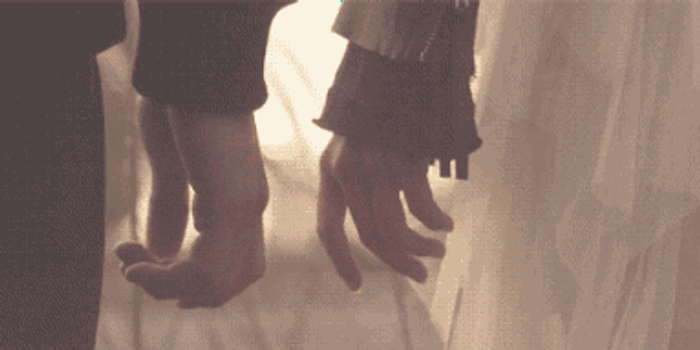## 新浪星座# 12星座负心男：分手的一百万个可能(组图)=白羊座=

=金牛座=

=双子座=

=巨蟹座=

=狮子座=

=处女座=

=天秤座=

=天蝎座=

=射手座=

=魔羯座=

=水瓶座=

=双鱼座=